## An electron in an electron gun is accelerated from rest by a potential of 25 kV applied over a distance of 1 cm. The final velocity o

Question

An electron in an electron gun is accelerated from rest by a potential of 25 kV applied over a distance of 1 cm.
The final velocity of the electrons is _____.
The mass of the electron is 9.1×10^(-31) kg and its charge is 1.6×10^(-19) C.

in progress 0
2 months 2021-07-31T16:48:38+00:00 1 Answers 5 views 0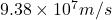Explanation:

We are given that

Potential ,V=25 kV=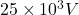Distance,r =1 cm=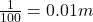1 m=100 cm

Mass of electron, m=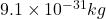Charge, q=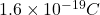We have to find the final velocity of the electron.

Speed of electron,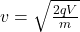Using the formula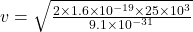v=Hence, the final velocity of the electron=